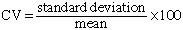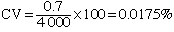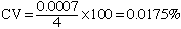I have a question from my OAC finite class. I've come across a problem with the coefficient of variation. I have taught my students that there are no units for coefficient of variation and it can be expressed as a percent. So, for example, a set of data with mean of 5 and standard deviation of 100 would have a CV of 5%. But what happens in this situation: the mean is 4meters and the standard deviation is 0.7mm. Is the CV 1.75% or 0.00175% or 0.0175%? I've had some students change leave the units as is, change them both to mm or change them both to meters...so which is it and why? thanks, Carmen teacher Hi Carmen, The coefficient of variation as a percent isIn your example you have it inverted. If the mean is 100 and the standard deviation is 5 then the coefficient of variation is 5%. You are quite right that the coefficient of variation is unit free, but only if the mean and standard deviation are measured in the same units. With a mean of 4 meters and a standard deviation of 0.7 millimeters you should convert the units of one of the quantities so that both are in the same units. To express both quantities in millimeters, 4 meters is 4 x 1000 = 4 000 millimeters and thus the coefficient of variation isYou could also express both quantities in meters. In this case 0.7 millimeters is  0.7/1000 = 0.0007 meters and the coefficient of variation isCheers, Penny Go to Math Central